# Topic 2: Mechanics

See the guide for this topic.

## 2.1 – Motion

• ### Distance and displacement

 Distance Displacement Scalar Vector A scalar quantity which measures how far two locations are apart from each other along a certain path. A vector quantity defined by the length and direction of the line segment joining the initial and final positions of an object.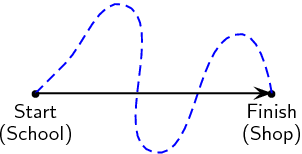• ### Speed and velocity

 Speed Velocity Scalar Vector Rate of change of distance to time. Rate of change of displacement to time.

Velocity is a measure dependent on the motion of the observer. The relative velocity of A to B is equal to the vector subtraction of the velocity of B from the velocity of A.

• ### Acceleration

 Acceleration Vector Rate of change of velocity

Acceleration due to gravity of any free-falling object is given by g=9.81m/s^2. This value does not depend on the mass of the object.

Take note that acceleration is a vector and thus has a direction. If we assume the upwards direction to be positive, the acceleration due to gravity would have a negative value of g=-9.81m/s^2.

### Displacement-time graph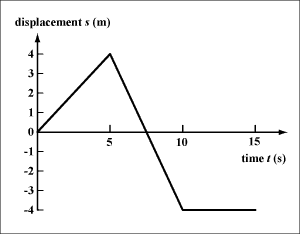The slope gradient indicates the velocity.

Straight lines imply constant velocity.

### Velocity-time graph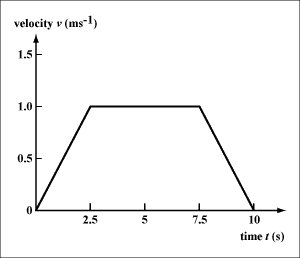The slope gradient indicates the acceleration.

Straight slanted lines imply constant acceleration or deceleration.

The area under the lines indicates the change in displacement.

### Acceleration-time graph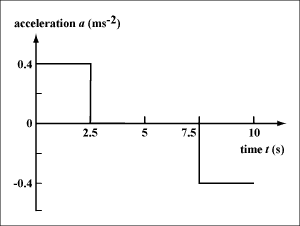Horizontal lines imply constant acceleration.

The area under the lines indicates the change in velocity.

• ### Equations of motion for uniform acceleration

 s = displacement u = initial velocity v = final velocity a = acceleration t = time taken

If acceleration is constant (uniform), the following equations can be used• ### Projectile motion

An object is said to undergo projectile motion when it follows a curved path due to the influence of gravity.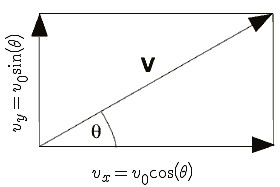If we assume air resistance to be negligible in a projectile motion:

• The horizontal component of velocity is constant
• The vertical component of velocity accelerates downwards at 9.81m/s^2
• The projectile reaches its maximum height when its vertical velocity is zero
• The trajectory is symmetricThe presence of air resistance changes the trajectory of the projectile by the following

• The maximum height of the projectile is lower
• The range of the projectile is shorter
• The trajectory is not symmetric
• ### Fluid resistance and terminal speed

Air resistance limits the maximum velocity an object could attain from free-falling. For example:

• If you jump out of a plane and undergo free-falling, you will feel an upward force exerted on you by the surrounding air due to air resistance.
• As you fall faster and faster due to gravity, this upward force exerted by air becomes greater and greater until it balances your weight. At this point, the net force acting on you becomes zero, and you no longer accelerate.
• This specific velocity at which you stop accelerating during a free-fall is called the terminal velocity.## 2.2 – Forces

• ### Objects as point particles

Forces change the velocity or shape of objects.

The unit of force is newton (N).

Objects are represented as a point mass to enable the representation for forces as arrows in free-body diagrams.

• ### Free-body diagrams

On a free body diagram, forces acting on an object are represented as arrows which stem from a point mass.

The length and direction of the arrows corresponds to the magnitude and the direction of the forces acting on the body of interest.Determining the resultant force

 1. Resolve all acting forces into horizontal and vertical components 2. Add up the horizontal components 3. Add up the vertical components 4. Combine the sum of horizontal components and the sum of vertical components
• ### Translational equilibrium

A body is said to be in translational equilibrium if it the net force acting on the body is zero. This means the body is either at rest or travels at constant velocity. For example:

• Mass hanging at rest
• Elevator moving upwards at constant velocity
• Parachutist reaching terminal velocity
• ### Newton’s laws of motion

Newton’s First Law (Law of Inertia) states that a body remains at rest or travels with constant speed along a straight line unless acted upon by an external force. (Net force = 0)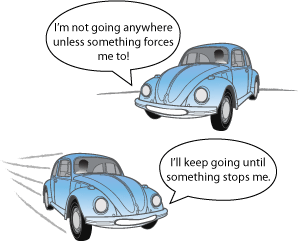Newton’s Second Law states that net force is directly proportional to acceleration and to mass. (F=ma)Newton’s Third Law states that if a body A exerts a force on body B, then body B exerts a force of the same magnitude but in the opposite direction of body A.

This pair of forces is called an action-reaction pair, which must act on two different bodies.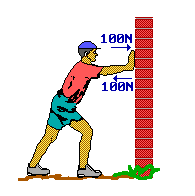• ### Solid friction

Friction is a non conservative force which opposes motion. If there is no motion, then there will be no force caused by friction.

For two solid surfaces moving over each other, the friction will be affected by the nature (roughness etc) of the two surfaces. However, the surface area and velocity of the object does not affect the friction.

There are also two types of friction for solid surfaces: static friction and kinetic friction. Static friction is that which stops objects from beginning to move. Kinetic friction is that which slows objects down when they are moving. Static friction is always larger than kinetic friction.

These two types of friction are defined individually by their constants µs and µk respectively.

The forces of friction are also dependent on the normal force the surface is applying, leading to Friction force (static) =< µs * Normal force for objects that are not moving and Friction force (static) = µs * Normal force for objects that are moving.## ​2.3 – Work, energy and power

• ### Kinetic energy

Kinetic energy (KE) is the energy of a body due to its motion and is given by the equation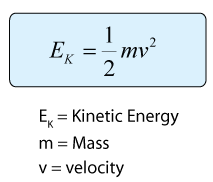• ### Gravitational potential energy

The gravitational potential energy (GPE) of an object changes with its height and is given by the equation• ### Elastic potential energy

Elastic energy is potential energy stored as a result of the deformation of an elastic object such as the stretching of a spring and is given by the equation• ### Work done as energy transfer

Work done measures the transfer of energy due to a force and is a scalar quantity.

The work done W by a force F on an object is given by the equationIn a force-displacement graph, work done is the area under the curve.• ### Power as rate of energy transfer

Power (P) is the work done or the energy output per time given by the equation: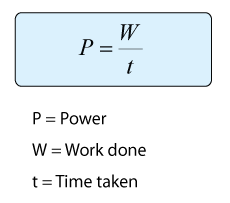For constant force acting on an object with constant velocity, the power is given by the equation: P=Fv.

• ### Principle of conservation of energy

Energy can neither be created nor destroyed; it can only be changed from one form to another. For example:

• An electrical heater transforms electrical energy to thermal energy.
• A falling object transforms potential energy to kinetic energy.

Total energy of an isolated body remains constant. In other words, ΔKE+ΔPE=0

• ### Efficiency

Efficiency is the ratio of useful energy output to energy input as a percentage given by the equation

## ​2.4 – Momentum and impulse

• ### Newton’s second law expressed in terms of rate of change of momentum

The linear momentum (p) is given by the equationThe linear momentum (p) is a vector with the same direction as the velocity of an object.

The change of momentum of an object is called impulse.

Rearranging the formula describing Newton’s second law results in the following expression• ### Impulse and force–time graphs

Impulse is given by the area of a force-time graph.• ### Conservation of linear momentum

The law of conservation of linear momentum states that the sum of initial momentum is equal to the sum of final momentum in a closed system and can be given by the equation

##• ### Elastic collisions, inelastic collisions and explosions

 Type Total momentum Total kinetic energy Elastic Conserved Conserved Inelastic Conserved Not conserved Explosion Conserved Not conserved